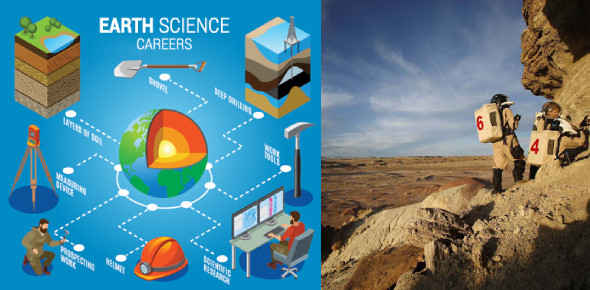# Earth Science And Geological History Quiz!

10 Questions | Total Attempts: 631SettingsCreate your own QuizEarth Science is the scientific study of the Earth and its surrounding in space. Earth scientists use their knowledge and skills to detect and research energy and mineral resources. This quiz will test your understanding of scientific facts about Earth and its history. Let's try it out. All the best!

• 1.
The area of land from which a stream or river collects runoff.
• A.

Mississippi River

• B.

Drainage Basin

• C.

Gully Erosion

• D.

Sheet Rock

• 2.
Mature Streams carve broad, flat, valley doors called:
• A.

Plains

• B.

Slowly

• C.

Floodplains

• D.

Plains

• 3.
Which of the following best describes how scientists classify different rocks?
• A.

By their shape

• B.

By how they are formed

• C.

By where they are found

• D.

All of the above

• E.

None of the Above

• 4.
What’s Earth’s true shape?
• A.

Oblate Spheroid

• B.

Flat

• C.

Sphere

• D.

Rectangle

• 5.
2. 67,000 mph (107,826 km/h) represents what speed?
• A.

Earth's orbit around the sun

• B.

Earth's movement through the milky way

• C.

Earth's rotation about its axis

• D.

None

• 6.
How much of the Earth's surface is covered with water?
• A.

25%

• B.

35%

• C.

50%

• D.

71%

• 7.
Roughly how old is Earth?
• A.

4.54 billion years

• B.

6,000 years

• C.

4.54 million years

• D.

2000 years

• 8.
Minus 100 degrees Fahrenheit (minus 73 degrees C) was the coldest temperature measured on Earth. Where?
• A.

The North Pole

• B.

• C.

Vostok Station, Antartica

• D.

Florida

• 9.
Since the early 19th century, which of these has moved northward more than 600 miles (1,100 kilometers)?
• A.

Earth's magnetic North Pole

• B.

New York City

• C.

The Equator

• D.

The Axis

• 10.
Roughly how often does the lightning strike somewhere on Earth?
• A.

100 times per day

• B.

100 times per second

• C.

100 times per hour

• D.

1000 times per  day

Related TopicsBack to top
×

Wait!
Here's an interesting quiz for you.